# CSVTU Exam Papers – BE I Year – Engineering Mechanics– April-May-2010

BE (1st year)

Examination, April-May-2010

Engineering Mechanics

1. (a) State Lami’s theorem ?

(b) A boat B, as shown in Fig. 1, is in the middle of a canal 100 m wide^ and is pulled through two ropes BA (150 m long) and BC (1,00 m long) by two men on the banks. The pull in BC = P = 150 N. Find the pull Q in BA so that the boat moves parallel to the banks. Find the resultant pull on the boat.

Fig:-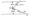(c) Two identical rollers each of weight Q = 100 N are supported by an inclined plane and a vertical wall as shown in Fig. 2. Assuming smooth surfaces, find the reactions at A, B, & C.

Fig:-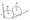(d) A loading car, as shown in Fig. 3, is at rest on a track formi an arjgle of 25° with the vertical. The gross weight of the cj & its load is 2^500 N .and it is applied at a point 75 cm from the track, cable attached 60 cm from the trai Determine the tension in the cable & reaction at each pair wheels. Neglect frictions.

Fig:-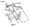Q.2 (a) Define point of contraflexure.

(b) Draw the shear force and bending moment diagrE for the beam as shown in Fig. 4.

Fig:-(c) A truss is loaded as shown in Fig. “5. The span of truss is m . Find out the forces in all the members of “the truss.

Fig:-(d) A truss is loaded and supported as shown in Fig. 6. Determine the axial forces in the members CE, CG & FG.

Fig:-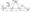Q.3.(a) Define limiting friction.

(b) What is the value of P in the system shown in Fig. 7 to cause the motion to impend ? Assume the pulley is smooth’& coefficient of friction between the other contact surfaces is 0.20.

Fig:-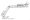(c) A ladder of length 4 – m weighing 200 N is placed against a vertical wall as shown in Fig, 8. The coefficient of friction between the wall & the ladder is 0.2 & that between the floor & the ladder is 0.3. The ladder in addition to its own weight has to support a man weighing 600 N at a distance, of 3 m from A. Calculate the minimum horizontal force to be applied at A to prevents slipping .

Fig:-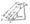(d) A belt 100 mm x 10 mm thick is transmittin power at 1200 m/min. The net driving tension is 1.8 times the tension on the slack side. If the safe stress on the belt section is 1.8 N/mm2 calculate the power that can be transmitted at this speed. Assume mass density of the leather as 1 t/m3. Also calculate the absolute maximum power that can be transmitted by this belt & the speed at which this can be transmitted.

Q.4.(a) State product of inertia.

(b) P rove that the moment of inertia of the area of a rectangle as shown in Fig. 9 about its diagonal is given by,

I=a3b3  /6(a2 + b2)

Fig:-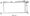(c) Determine the mass moment of inertia of a unit length L about its centroidal axis normal to rod.

(d) For a z—section as shown in Fig. 10, the moment of inertia with respect to the x and y axes are given to be  lx = 154 axes of cm4 and ly = 2668 cm4 Determine the principal axes of section about 0 and the values of the principal inertia.

Fig:-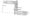Q.5.(a) Explain D’Alembert’s principle.

(b) Two weights 800 N and 200 N are connected bl and they move along a rough horizontal plane action ®f a ‘force of 400 – N appljed to the 800 N shown in Fig. 11. The coefficient of friction be sliding surface of the weights & the plane is D’Alembert’s principle determine the acceleration tension in thread.

Fig:-(c) Determine the constant force P that will give the system bodies shown in>Fig. 12 a velocity of 3 m/s after moving 4m from rest. Coefficient of friction between the-blocks & the plane is 0.3. Pulleys are smooth.

Fig:-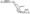(d) A glass marbel, whose weight is 0.2 N1 falls from a height c 10 m and rebounds to a height of 8 metres. Find the impuls and the average force between the marble and the floor, the time during which they are in contact is 1/10 of a second.